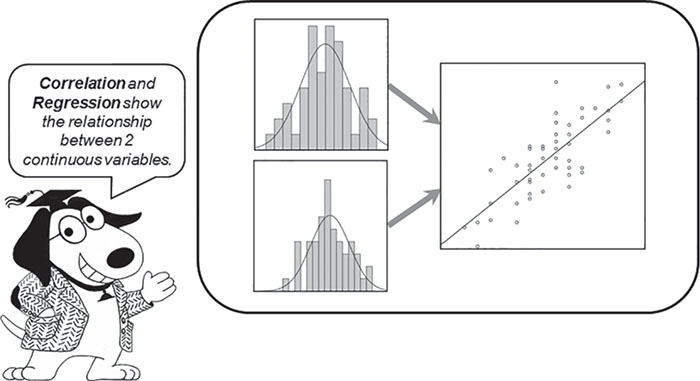What statistical test should I use for this kind of data? How do I set up the data? What parameters should I specify when ordering the test? How do I interpret the results? Herschel Knapp’s friendly and approachable guide to real-world statistics answers these questions. Intermediate Statistics Using SPSS is not about abstract statistical theory or the derivation or memorization of statistical formulas–it is about applied statistics. With jargon-free language and clear processing instructions, this text covers the most common statistical functions–from basic to more advanced. Practical exercises at the conclusion of each chapter offer students an opportunity to process viable data sets, write cohesive abstracts in APA style, and build a thorough comprehension of the statistical process. Students will learn by doing with this truly practical approach to statistics. Free downloadable tutorial videos provide an overview of each statistical method!

# Correlation and Regression: Pearson and Spearman

### Correlation and Regression: Pearson and SpearmanHe who laughs most, learns best.

John Cleese

### Learning Objectives

Upon completing this chapter, you will be able to do the following:

• Determine when it is appropriate to run Pearson regression and Spearman correlational analyses.
• Interpret the direction and strength of a correlation.
• Verify that the data meet the criteria for running regression and correlational analyses: normality, linearity, and homoscedasticity.
• Order a regression analysis: correlation and scatterplot with regression line.
• Interpret the test results.
• Resolve the hypotheses.
• Write an appropriate abstract.
• Understand the criteria for causation: association/correlation, temporality, and nonspurious.
• Differentiate between correlation and causation

### When to Use This Statistic### Guidelines for Selecting the Pearson Test

Overview: This statistic indicates if there is a correlation between two continuous variables.

Variables: This statistic requires two continuous variables for each record.

Results: To determine if there ...

•••